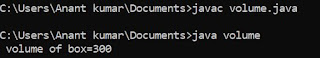## Tuesday, 14 August 2018

### What is recursion in C ?

When a function call to itself or when a function call to a second function and in function definition of second function again a function call statement for first function is encountered, it is known as recursion and this types of function is called recursive function.

### Types of recursion in C ?

1. Direct recursion :

ABC ()
{
"
"
"
ABC () ;
}

2. Indirect recursion :

ABC ()
{
"
XYZ () ;
"
XYZ ()
{
"
ABC () ;
"

### Program of recursive function to find factorial in C:

#include < stdio.h>
int factorial ( int ) ;
void main ()
{
int a , b ;
printf ("\n Enter a number:") ;
scanf ("%d", &a) ;
b = factorial (a) ;
printf ("%d" , b) ;
getch () ;
}
int factorial ( int a )
{
if ( a == 0 )
return 1 ;
else
return ( a * factorial ( a - 1 ) ) ;
}